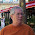VHDL coding tips and tricks: VHDL code for BCD to Binary conversion

Monday, April 27, 2015

VHDL code for BCD to Binary conversion

In the past I have posted various codes for Binary to BCD conversion, BCD addition, BCD seven segement display etc. And I got few queries for binary to bcd conversion. So here it is:

The input is 4 digit BCD's, each 4 bit in size. And the output is 14 bit binary. The input can range from 0000 to 9999 in decimal.

With few changes in the code, it can be extended for more number of BCD digits. I hope you will experiment with the code to see how it works. Have fun!

VHDL Code:

library IEEE;
use IEEE.STD_LOGIC_1164.ALL;
use IEEE.STD_LOGIC_ARITH.ALL;
use IEEE.STD_LOGIC_UNSIGNED.ALL;

--this module is for converting a 4 digit BCD number into binary number.
--the range of the input in decimal is 0 to 9999.
entity bcd_2_bin is
Port ( bcd_in_0 : in  STD_LOGIC_VECTOR (3 downto 0);
bcd_in_10 : in  STD_LOGIC_VECTOR (3 downto 0);
bcd_in_100 : in  STD_LOGIC_VECTOR (3 downto 0);
bcd_in_1000 : in  STD_LOGIC_VECTOR (3 downto 0);
bin_out : out  STD_LOGIC_VECTOR (13 downto 0) := (others => '0'));
end bcd_2_bin;

architecture Behavioral of bcd_2_bin is

begin

bin_out <= (bcd_in_0 * "01")  --multiply by 1
+ (bcd_in_10 * "1010") --multiply by 10
+ (bcd_in_100 * "1100100") --multiply by 100
+ (bcd_in_1000 * "1111101000"); --multiply by 1000

end Behavioral;

Testbench Code:

LIBRARY ieee;
USE ieee.std_logic_1164.ALL;

ENTITY tb_bb IS
END tb_bb;

ARCHITECTURE behavior OF tb_bb IS

COMPONENT bcd_2_bin
PORT(
bcd_in_0 : IN  std_logic_vector(3 downto 0);
bcd_in_10 : IN  std_logic_vector(3 downto 0);
bcd_in_100 : IN  std_logic_vector(3 downto 0);
bcd_in_1000 : IN  std_logic_vector(3 downto 0);
bin_out : OUT  std_logic_vector(13 downto 0)
);
END COMPONENT;

signal bcd_in_0 : std_logic_vector(3 downto 0) := (others => '0');
signal bcd_in_10 : std_logic_vector(3 downto 0) := (others => '0');
signal bcd_in_100 : std_logic_vector(3 downto 0) := (others => '0');
signal bcd_in_1000 : std_logic_vector(3 downto 0) := (others => '0');
signal bin_out : std_logic_vector(13 downto 0);

BEGIN

-- Instantiate the Unit Under Test (UUT)
uut: bcd_2_bin PORT MAP (
bcd_in_0 => bcd_in_0,
bcd_in_10 => bcd_in_10,
bcd_in_100 => bcd_in_100,
bcd_in_1000 => bcd_in_1000,
bin_out => bin_out
);

-- Stimulus process
stim_proc: process
begin
bcd_in_0 <= x"0"; bcd_in_10 <= x"1"; bcd_in_100 <= x"2"; bcd_in_1000 <= x"3";
wait for 100 ns;
bcd_in_0 <= x"9"; bcd_in_10 <= x"9"; bcd_in_100 <= x"9"; bcd_in_1000 <= x"9";
wait for 100 ns;
bcd_in_0 <= x"9"; bcd_in_10 <= x"2"; bcd_in_100 <= x"4"; bcd_in_1000 <= x"6";
wait for 100 ns;
bcd_in_0 <= x"0"; bcd_in_10 <= x"0"; bcd_in_100 <= x"0"; bcd_in_1000 <= x"0";
wait;
end process;

END;

Simulation waveform:

The code was simulated using Xilinx ISIM.

1.2.3.